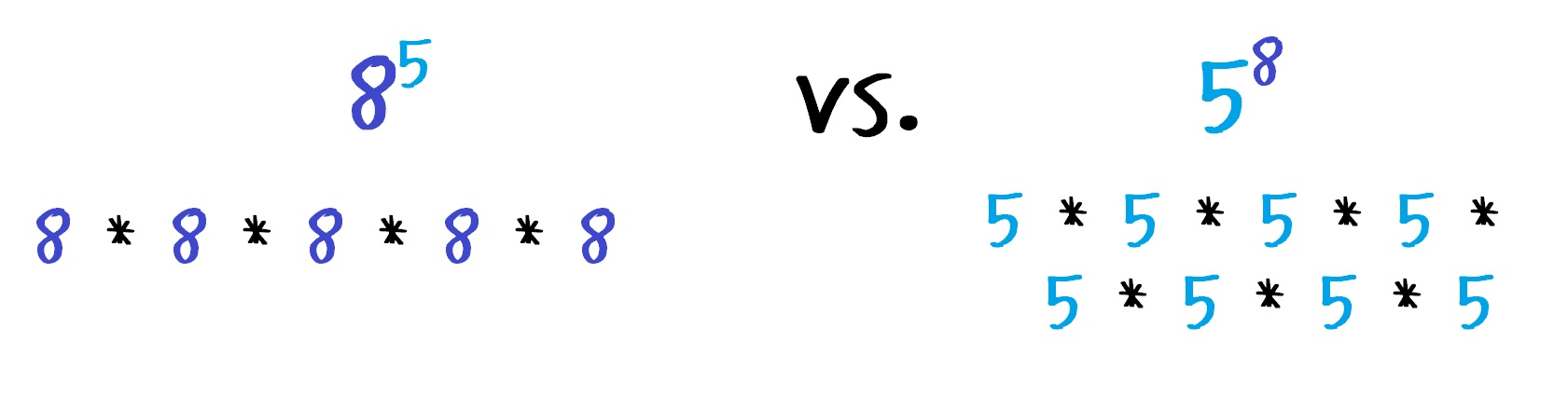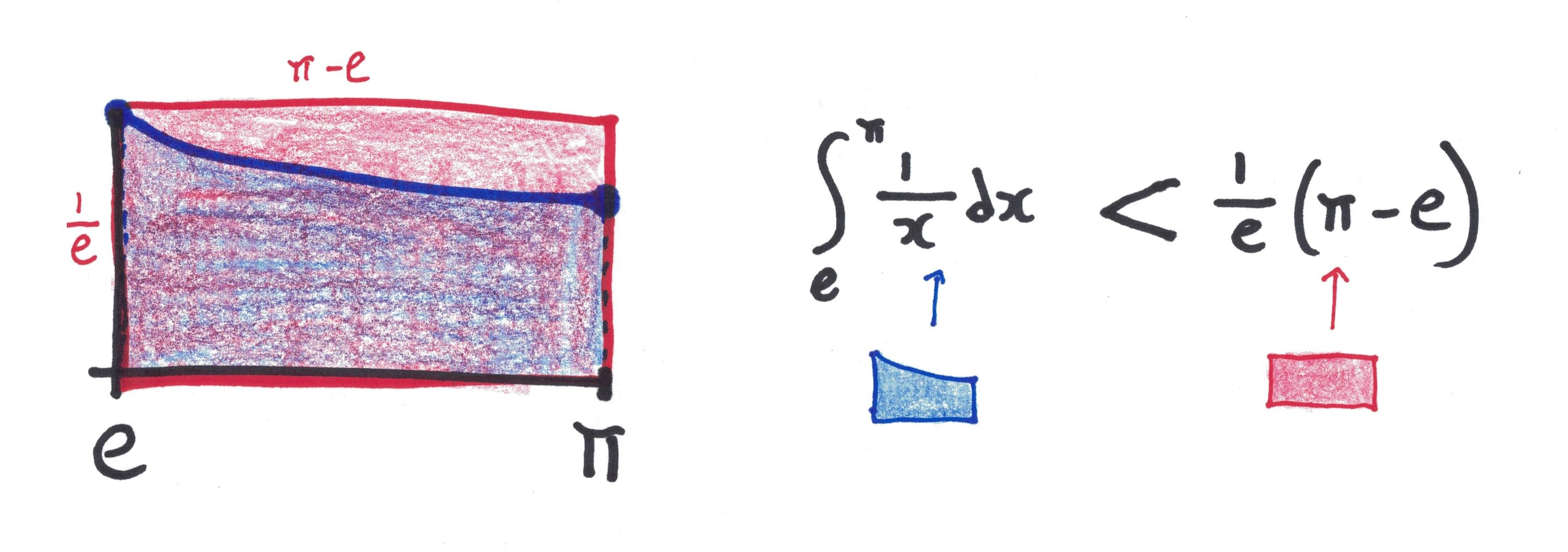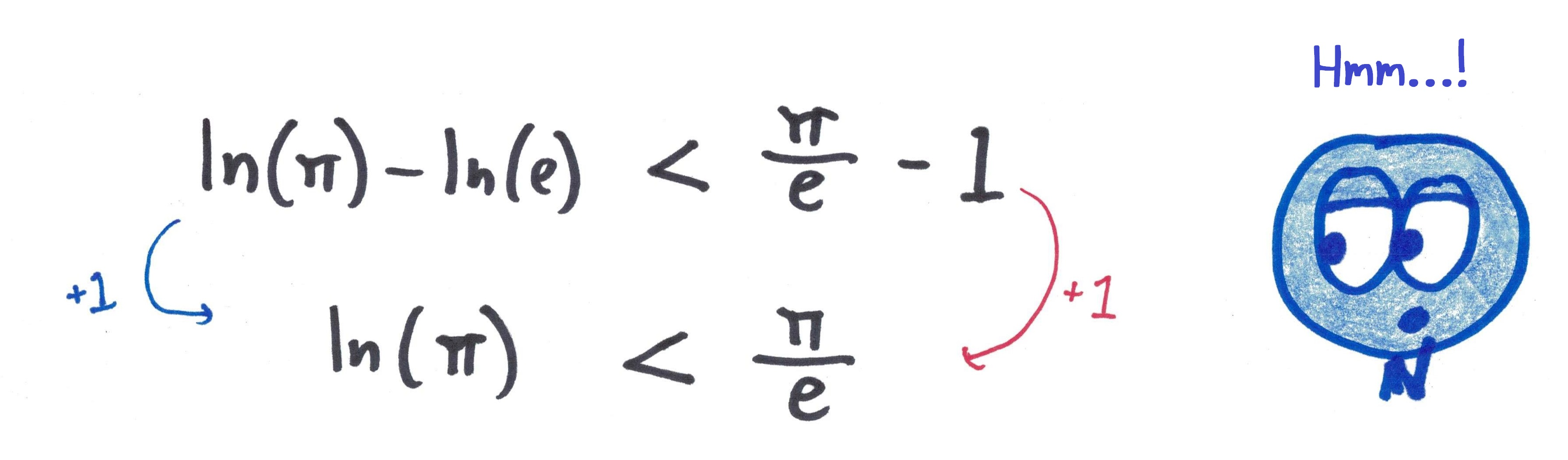# Powers Great and Small

I was not always a droop-shouldered 30-year-old. Once upon a time, I was a bright-eyed 29-year-old with my life still before me—and back then, I used to ply my 7th-graders with a puzzle I called SMALLBIG vs. BIGSMALL.Like “Dude, Where’s My Car?” or “Who’s Afraid of Virginia Woolf?,” the question is pretty much there in the title. You’ve got two numbers—say, 5 and 8. The question is, which will yield a larger result: using the larger number as an exponent (e.g., 58) or using it as a base (e.g., 85)?

It’s a choice between more, smaller factors vs. fewer, bigger factors.I thought the question was smoking hot. But the lessons fizzled like dud fireworks. Kids would merrily plug away with their calculators, filling out tables of integer values. They’d find that SMALLBIG almost always defeated BIGSMALL. They’d note the odd exceptions—that 1n is always less than n1, and that 32 somehow outcompetes 23, and that 42 equals 24.

And then they’d shrug and say, “What’s next?”

My mind would spark with quicksilver connections. There’s so much here! I’d think. The sneaky strength of exponentiation! The construction of Graham’s number! The dominance of the largest-degree term in a polynomial as x goes to infinity!

But I could never get the fire to catch. Not even in my own brain.

To explain, I might say: Introducing more factors outweighs making the factors a little bigger—but that’s not an explanation, just a paraphrase of the pattern. Or perhaps: Exponentiation is repeated multiplication, and the real power lies in the repetition—nope, that’s just another paraphrase, with an extra scoop of vagueness. Or even: Think about the difference between 1002 and 2100—which is an illustrative example, sure, but it doesn’t explain the rule.

What I learned: It’s hard to elicit crisp, decisive reasoning from the students when you can’t even achieve it yourself.Years pass. 7th-graders become 8th-graders, who become 9th graders, who become inscrutable eleven-foot beings with voices like bass trombones. And one evening, I find myself struck by a tweet from Luca Moroni, highlighting a lovely visual proof that eπ is bigger than πe.

To unpack the logic—and if calculus is not your preferred sandwich spread, feel free to skip down—we set up an integral, inspecting the area under the curve y = 1/x:Then, we note that this area must be smaller than the area of the rectangle that encloses it:Rewriting the integral (via the Fundamental Theorem of Calculus), we get:Evaluating ln(e) as 1 and adding 1 to both sides, we have:And then, applying some nifty logarithmic rules, we conclude:You can verify the numbers for yourself: eπ is 23.141ish, whereas πe is 22.459ish. That’s a pretty small difference! I love that a geometric, calculus-based argument can cleave the two apart.

The mathematical temperature rose even higher when a teacher named Dirk L. made an astute point: This isn’t anything special about π. The same argument shows ea > ae for all a greater than e.Thoughts now came to me in quick succession:

1. That’s really cool!
2. This is exactly what my activity with the 7th-graders was getting at.
3. I didn’t understand the math well enough to teach that lesson properly.
4. I should put some clothes on. (I’d just gotten out of the shower.)

Freshly clad, I realized Dirk’s observation had another easy corollary: ea > ae for all a smaller than e. For example e2 > 2e.In other words: enumber > numbere for literally all positive numbers, whether bigger or smaller than e. And, necessarily, e is the unique number with this property.

It is—as they say—the “natural base.”

I think this fact is desperately cool. I’m talking glacier-cool, Lando-cool, as cool as living through the Golden Age of Seven-Track Albums Produced by Kanye West. And yet, when I wrote a celebratory e day post earlier this year, my scrounging didn’t turn up this fact. This baffles me! I want to sing this fact from the rooftops.What’s the takeaway?

Well, on a mathematical level: continuity is glorious and necessary.

Looking only at the integers, the SMALLBIG vs. BIGSMALL question is a hopeless mishmash, all heuristics and exceptions. Clearly something important happens between 2 and 3. But without delving into the real number line, you’re at a loss to explain where, or why.

The mathematical process of generalization often freaks people out. What does 7e even mean? But we do it for a reason: by enlarging the scope of our theories, we can achieve otherwise impossible levels of clarity and unity.

A second fundamental lesson—one I’ve learned a hundred times and will no doubt learn a thousand more—is that I’ve always got more to learn.

Don’t forget: I liked the SMALLBIG vs. BIGSMALL question precisely because it struck me as deep. But it ran even deeper than I guessed, deeper than the Dead Sea. Comparing integral powers—611 vs. 116—doesn’t sound too fancy. But it slides naturally into questions of continuity, irrationality, logarithms, the approximation of integrals…

I sometimes think that there are no puddles in math; there are only oceans in disguise.## 15 thoughts on “Powers Great and Small”

1.jimorlin says:

Here is another way to see that e^x > x^e for all x not equal to e. Let f(x) = e^x. Let g(x) = x^e. Note that f(e) = g(e). Now take the derivatives. Then f'(x) = e(x). g'(x) = e x^(e-1), For x > e, f'(x) > g'(x). For x < e, f'(x) g(x) if x is not e.

1.Ben Orlin says:

Ah, thanks – much more natural than the integral-magic-trick proof in the post! I like ’em both.

2.maksimm says:

An extension of this to address the original $a^b ? b^a$. We take log: $b \ln a ? a \ln b$$,$\frac{\ln a}{a} ? \frac{\ln b}{b}$. We introduce$f(x)=\frac{\ln x}{x}$and study its behavior; taking derivative we see that it is maximal at$x=e$, so$e^x>x^e$always for$x\neq e$. We also notice that$f(2)=f(4)$so$2^4=4^2$, and$f(3)>f(2)$which is “why”$3^2>2^3$. Of course$f(1)=01$so$1^xb$implies$f(a) a^b\$, which is to say that the “odd exceptions” are the only ones.

1.maksimm says:

I apologize — I’m too used to stackexchange with both LaTeX-like syntax and ability to edit comments. If you get a chance to edit this so it is somewhat more WordPress readable, please do so.

3.maksimm says:

An extension of this to address the original a^b ? b^a. We take log: b ln a ? a \ln b, or ln a/a ? ln b/b. We introduce f(x)=ln x/x and study its behavior; taking derivative we see that it is maximal at x=e, so e^x>x^e for all x not equal to e. We also notice that f(2)=f(4) so 2^4=4^2, and f(3)>f(2) which is “why” 3^2>2^3. Of course f(x)> f(1)=0 for all x>1 so x^1>1^x ; for all other positive integer a and b, a>b implies f(b)>f(a), which is to say that the “odd exceptions” are the only ones.

2.gregsdennis says:

As an object-oriented software developer I like to adhere to the Single Responsibly Principle that states any unit of coffee should have but one purpose. In that regard, *e* does to much and should be banned. It’s a mathematical monopoly and should be split. Let’s not quibble over how to accomplish this; instead, blindly join me in my fight against the dangers of *e*!

1.gregsdennis says:

Stupid phone autocorrect… “coffee” should be “code”

1.Doug M says:

I liked the comment being about coffee.

What is the one purpose? To wake us up, or are there people who really do like the taste?

Or each “unit” of coffee can have its own purpose depending on the time of day, location, corporate grind or boutique… (but coffee is liquid, does it have a unit?)

1.gregsdennis says:

Nice. Fighting sarcasm with sarcasm.

3.the 14-year-old that just outsmarted you says:

Not bad. Except I’m a geometry person, so I like the other one better. Sorry!

4.Rudolf Polzer says:

I once wondered about that too, and my own resolution was (excluding 0 for now):

a^b b^a | take the ab-th root
a^(1/a) b^(1/b)

Suddenly it’s a property about the numbers themselves. No more formula involving both. So what is f(x) = x^(1/x)?

Clearly for x -> +infinity this is 1. Just like for x = 1. And for x -> +0 it becomes zero.

Taking the logarithm might make it easier – we then have (ln x) / x. Derivative? (1 – ln x) / x^2. Very nice. Positive left of e, negative right of e. So the maximal value is achieved at e, making e the “best base”.

So we can now partially answer the question: a^b > b^a if a and b are “on the same side of e” and a is closer to e than b. As for flipping the side of e – that is actually hard. I could not find an analytic way to find pairs like 2, 4 so that (ln a) / a = (ln b) / b, i.e. a^b = b^a. But it does mean that for the purpose of the above “shorthand” to answer this, 2 and 4 are “equally close to e”. As the other known such pair is (1, infinity), we already know all we need to answer a^b = b^a for positive integers (I even added the zero back that we previously excluded):

– Look for your numbers in this “sequence”: 3, (2 tied with 4), 5, 6, 7, 8, 9, …, 1, 0 (being the omega’th and (omega+1)th elements of this ordinal sequence).
– The number that comes first is the “better base”, i.e. the one yielding the larger result.

5.Vincent says:

This is great!